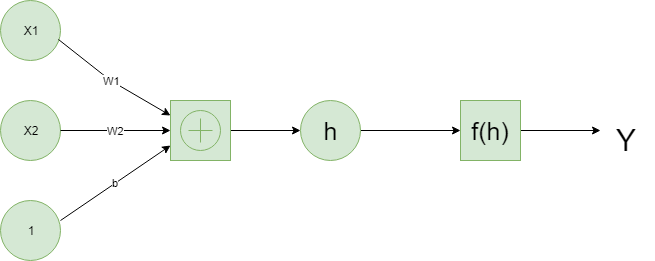# Deep Learning with PyTorch | An Introduction

• Difficulty Level : Medium
• Last Updated : 07 Feb, 2019

PyTorch in a lot of ways behaves like the arrays we love from Numpy. These Numpy arrays, after all, are just tensors. PyTorch takes these tensors and makes it simple to move them to GPUs for the faster processing needed when training neural networks. It also provides a module that automatically calculates gradients (for backpropagation) and another module specifically for building neural networks. All together, PyTorch ends up being more flexible with Python and the Numpy stack compared to TensorFlow and other frameworks.

Neural Networks:
Deep Learning is based on artificial neural networks which have been around in some form since the late 1950s. The networks are built from individual parts approximating neurons, typically called units or simply “neurons.” Each unit has some number of weighted inputs. These weighted inputs are summed together (a linear combination) then passed through an activation function to get the unit’s output.
Below is an example of a simple neural net.Tensors:
It turns out neural network computations are just a bunch of linear algebra operations on tensors, which are a generalization of matrices. A vector is a 1-dimensional tensor, a matrix is a 2-dimensional tensor, an array with three indices is a 3-dimensional tensor. The fundamental data structure for neural networks are tensors and PyTorch is built around tensors.

It’s time to explore how we can use PyTorch to build a simple neural network.

 `# First, import PyTorch ` `import` `torch `

Define an activation function(sigmoid) to compute the linear output

 `def` `activation(x): ` `    ``""" Sigmoid activation function  ` `     `  `        ``Arguments ` `        ``--------- ` `        ``x: torch.Tensor ` `    ``"""` `    ``return` `1``/``(``1` `+` `torch.exp(``-``x)) `

 `# Generate some data  ` `# Features are 3 random normal variables ` `features ``=` `torch.randn((``1``, ``5``)) ` ` `  `# True weights for our data, random normal variables again ` `weights ``=` `torch.randn_like(features) ` ` `  `# and a true bias term ` `bias ``=` `torch.randn((``1``, ``1``)) `

`features = torch.randn((1, 5))` creates a tensor with shape (1, 5), one row and five columns, that contains values randomly distributed according to the normal distribution with a mean of zero and standard deviation of one.

`weights = torch.randn_like(features)` creates another tensor with the same shape as features, again containing values from a normal distribution.

Finally, `bias = torch.randn((1, 1))` creates a single value from a normal distribution.

Now we calculate the output of the network using matrix multiplication.

 `y ``=` `activation(torch.mm(features, weights.view(``5``, ``1``)) ``+` `bias) `

That’s how we can calculate the output for a single neuron. The real power of this algorithm happens when you start stacking these individual units into layers and stacks of layers, into a network of neurons. The output of one layer of neurons becomes the input for the next layer. With multiple input units and output units, we now need to express the weights as a matrix.

We define the structure of neural network and initialize the weights and biases.

 `# Features are 3 random normal variables ` `features ``=` `torch.randn((``1``, ``3``)) ` ` `  `# Define the size of each layer in our network ` ` `  `# Number of input units, must match number of input features ` `n_input ``=` `features.shape[``1``]      ` `n_hidden ``=` `2`                `# Number of hidden units  ` `n_output ``=` `1`                `# Number of output units ` ` `  `# Weights for inputs to hidden layer ` `W1 ``=` `torch.randn(n_input, n_hidden) ` ` `  `# Weights for hidden layer to output layer ` `W2 ``=` `torch.randn(n_hidden, n_output) ` ` `  `# and bias terms for hidden and output layers ` `B1 ``=` `torch.randn((``1``, n_hidden)) ` `B2 ``=` `torch.randn((``1``, n_output)) `

Now we can calculate the output for this multi-layer network using the weights W1 & W2, and the biases, B1 & B2.

 `h ``=` `activation(torch.mm(features, W1) ``+` `B1) ` `output ``=` `activation(torch.mm(h, W2) ``+` `B2) ` `print``(output) `

My Personal Notes arrow_drop_up
Recommended Articles
Page :